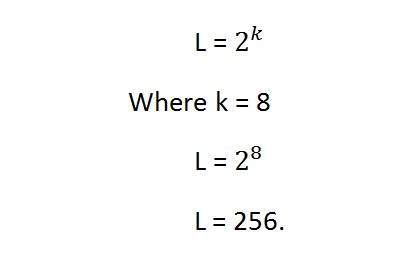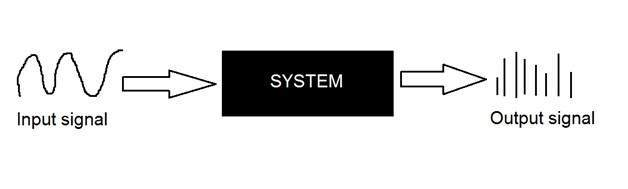# Gray Level Resolution

## Image resolution

A resolution can be defined as the total number of pixels in an image. This has been discussed in Image resolution. And we have also discussed, that clarity of an image does not depends on number of pixels, but on the spatial resolution of the image. This has been discussed in the spatial resolution. Here we are going to discuss another type of resolution which is called gray level resolution.

## Gray level resolution

Gray level resolution refers to the predictable or deterministic change in the shades or levels of gray in an image.

In short gray level resolution is equal to the number of bits per pixel.

We have already discussed bits per pixel in our tutorial of bits per pixel and image storage requirements. We will define bpp here briefly.

## BPP

The number of different colors in an image is depends on the depth of color or bits per pixel.

### Mathematically

The mathematical relation that can be established between gray level resolution and bits per pixel can be given as.In this equation L refers to number of gray levels. It can also be defined as the shades of gray. And k refers to bpp or bits per pixel. So the 2 raise to the power of bits per pixel is equal to the gray level resolution.

For example:The above image of Einstein is an gray scale image. Means it is an image with 8 bits per pixel or 8bpp.

Now if were to calculate the gray level resolution, here how we gonna do it.It means it gray level resolution is 256. Or in other way we can say that this image has 256 different shades of gray.

The more is the bits per pixel of an image, the more is its gray level resolution.

## Defining gray level resolution in terms of bpp

It is not necessary that a gray level resolution should only be defined in terms of levels. We can also define it in terms of bits per pixel.

### For example

If you are given an image of 4 bpp, and you are asked to calculate its gray level resolution. There are two answers to that question.

The first answer is 16 levels.

The second answer is 4 bits.

### Finding bpp from Gray level resolution

You can also find the bits per pixels from the given gray level resolution. For this, we just have to twist the formula a little.

Equation 1.This formula finds the levels. Now if we were to find the bits per pixel or in this case k, we will simply change it like this.

K = log base 2(L) Equation (2)

Because in the first equation the relationship between Levels (L ) and bits per pixel (k) is exponentional. Now we have to revert it, and thus the inverse of exponentional is log.

Lets take an example to find bits per pixel from gray level resolution.

### For example:

If you are given an image of 256 levels. What is the bits per pixel required for it.

Putting 256 in the equation, we get.

K = log base 2 ( 256)

K = 8.

So the answer is 8 bits per pixel.

## Gray level resolution and quantization:

The quantization will be formally introduced in the next tutorial, but here we are just going to explain the relation ship between gray level resolution and quantization.

Gray level resolution is found on the y axis of the signal. In the tutorial of Introduction to signals and system, we have studied that digitizing a an analog signal requires two steps. Sampling and quantization.Sampling is done on x axis. And quantization is done in Y axis.

So that means digitizing the gray level resolution of an image is done in quantization.